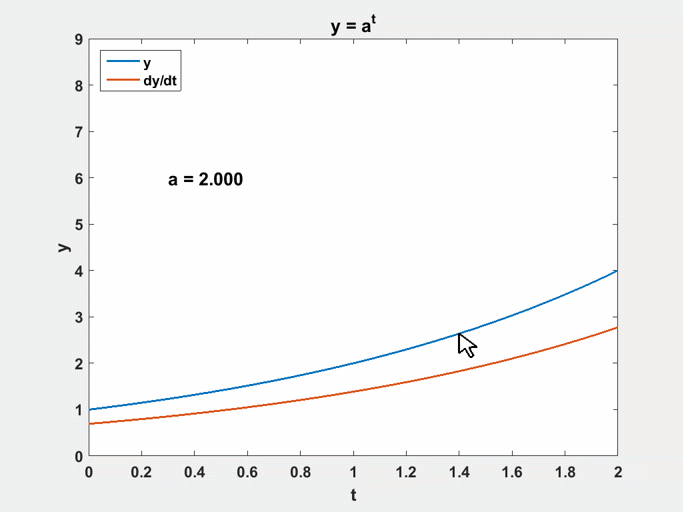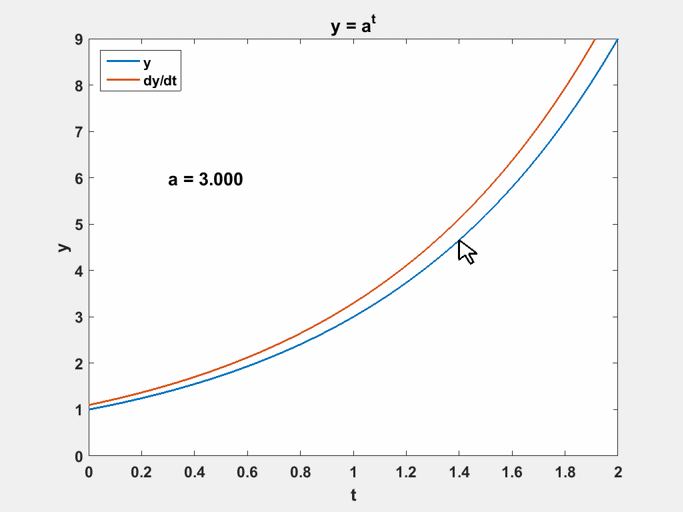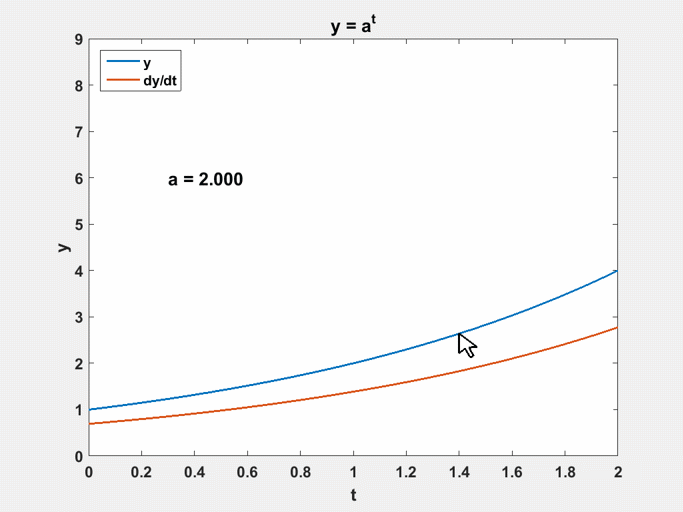# Discover e with a graphical experiment

An interactive graphical experiment lets you discover the value of one of the most important numerical quantities in mathematics.

### Contents

#### One of My Favorites

One of my favorite graphical experiments allows you to find the numerical value of $e$. The program is described in Chapter 8 of Experiments with MATLAB and is included in the toolbox available with that book. It was originally called expgui; today it is known as expshow. Here is a link to the code. I hope you are able to start up MATLAB and actually run expshow while you are reading this blog.

#### Approximate derivative

Let's say you've forgotten how to find the derivative of $a^t$ with respect to $t$. Be careful. Don't blindly follow your memory that the derivative of $t^n$ is $n t^{n-1}$ and claim that the derivative of $a^t$ is $t a^{t-1}$. For graphical purposes, we can use an approximate derivative, replacing the tangent by a secant with a step size of $10^{-4}$. Here is the core of expshow and the resulting screen shot with $a = 2$
   t = 0:1/64:2;
h = .0001;
% Compute y = a^t and its approximate derivative.
y = a.^t;
yp = (a.^(t+h) - a.^t)/h;#### Derivative of $2^t$ is below

The blue line is the graph of $2^t$. At $t = 1$ it passes through $y = 2$ and at $t = 2$ it hits $y = 4$. The sienna line is the graph of the derivative of $2^t$. The important facts are that it has the same shape as the blue line and lies entirely below it.

#### Derivative of $3^t$ is above

Now take your mouse and move the blue line. It's very satisfying to be actually interact with this experiment. Push the blue line until the sienna line moves above it, to somewhere around $a = 3$.With $a = 3$, at $t = 1$ the graph passes through $y = 3$ and at $t = 2$ it hits $y = 9$. The sienna line is now the graph of the derivative of $3^t$. The important facts are that it still has the same shape as the blue line and now lies entirely above it.

#### Action

Now you know what to do.Using the mouse, move the blue line until the two lines lie on top of each other. You have found the only function in the world that is equal to its own derivative and, in the process, discovered that, to three decimal places, the crucial value of the base is 2.718. And you did this without touching the keyboard or typing in any numbers.

Published with MATLAB® R2015a

|